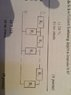# Infinite sequence of resistors

• diredragon

## Homework Statement

From the picture below, calculate the net resistance between points A and B if
##R_1=12##
##R_2=3.75##

## Homework Equations

3. The Attempt at a Solution [/B]
I cannot think of any way but to find the equivalent resistance od ##R_1## and ##R_2## and add them up but since there are infinite number of those equivalences the resistance at the end is infinity!
##R_{12}=\frac{R_1R_2}{R_1+R_2}= 2.86##
The infinite sequence od these gives inifinity so what is wrong?

#### Attachments

•image.jpg
28.9 KB · Views: 434

Mentor Note: members are reminded that on this forum the assistance given is to take the form of hints and guidance. Complete homework solutions do not help understanding and must not be contributed.

I cannot think of any way but
If you glance towards the foot of this page you will see links to some older PF threads which may cast light on the problem at hand.

•diredragon
Is this a multiple choice question? If so, what are the answers to choose from?

The choices are 15, 15.75, 2.86, 30, 7.5, infinity.
I found the equation ##R^2 - R_1R - R_1R_2=0## on the web and it came out with 15 so i think that is the answer

You can easily check your answer. Arrange 12, 3.75 and 15 Ω resistors and see what the result is.